# Acceleration is related to velocity and time by the following expression: a = vp tq

Acceleration is related to velocity and time by the following expression: a = vp tq. Find the powers p and q that make this equation dimensionally consistent.

The relation for acceleration in terms of velocity and time is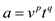Here, a is acceleration, v is velocity and t is time.
The dimensional formula for acceleration is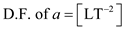The dimensional formula for velocity is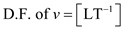The dimensional formula for time is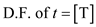SOLVE:
The powers and for the dimensional consistency of the given relation are found as follows.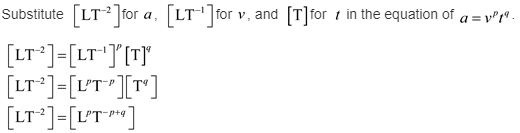Compare exponents of L on both sides of the above equation.Compare exponents of T on both sides of the above equation.Substitute 1 for q in the above equation.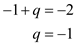Therefore, the powers p and q for the dimensional consistency of the given relation are
p = 1 and q = -1